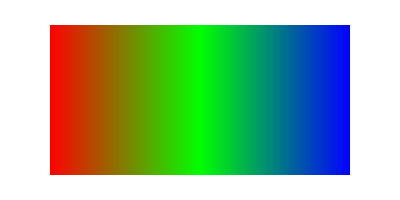VectorSourceLinearGradient()

Syntax

VectorSourceLinearGradient(x1.d, y1.d, x2.d, y2.d)
Description
Selects a linear color gradient as the source for vector drawing operations such as FillPath() or StrokePath(). Initially, the gradient is solid black. Color stops have to be added with the VectorSourceGradientColor() after this function.

Parameters

 x1.d, y1.d Specifies the point that represents the start (Position 0.0) of the gradient. The coordinates are specified in terms of the #PB_Coordinate_Source coordinate system. x2.d, y2.d Specifies the point that represents the end (Position 1.0) of the gradient. The coordinates are specified in terms of the #PB_Coordinate_Source coordinate system.

Return value

None.

Remarks

See the vectordrawing overview for an introduction to the different coordinate systems.

The color gradient is only defined in the area between the (x1, y1) and (x2, y2) points. Outside of these points, the used source color is depending on the operating system, so drawing operations outside of the defined gradient's area should be avoided.

Example

```  If OpenWindow(0, 0, 0, 400, 200, "VectorDrawing", #PB_Window_SystemMenu | #PB_Window_ScreenCentered)
CanvasGadget(0, 0, 0, 400, 200)

If StartVectorDrawing(CanvasVectorOutput(0))

VectorSourceLinearGradient(50, 0, 350, 0)
VectorSourceGradientColor(RGBA(255, 0, 0, 255), 0.0)
VectorSourceGradientColor(RGBA(0, 255, 0, 255), 0.5)
VectorSourceGradientColor(RGBA(0, 0, 255, 255), 1.0)

AddPathBox(50, 25, 300, 150)
FillPath()

StopVectorDrawing()
EndIf

Repeat
Event = WaitWindowEvent()
Until Event = #PB_Event_CloseWindow
EndIf
```See Also

VectorSourceGradientColor(), VectorSourceCircularGradient(), VectorSourceColor(), VectorSourceImage()

Supported OS

All

<- VectorSourceImage() - VectorDrawing Index - VectorTextHeight() ->